# Efficient Algoirthms for Routing Dependable Connections in WDM

• Slides: 37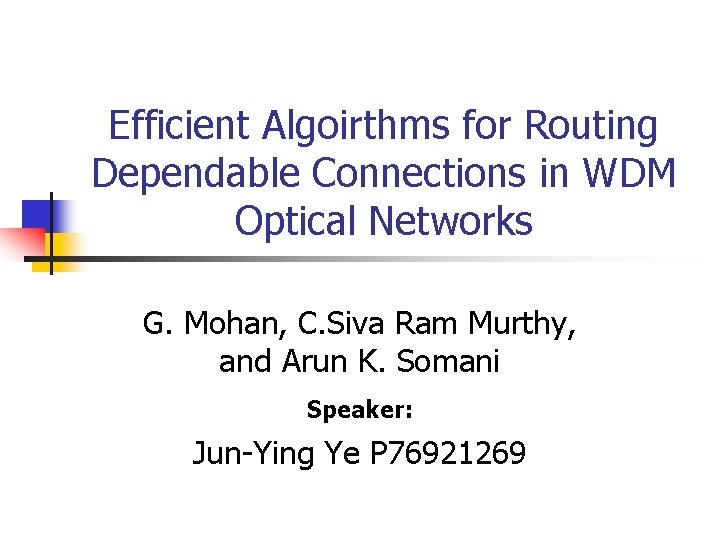Efficient Algoirthms for Routing Dependable Connections in WDM Optical Networks G. Mohan, C. Siva Ram Murthy, and Arun K. Somani Speaker: Jun-Ying Ye P 76921269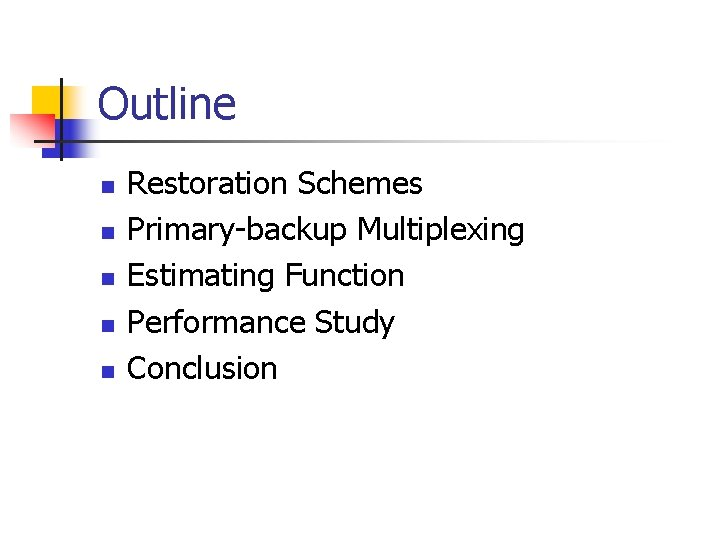Outline n n n Restoration Schemes Primary-backup Multiplexing Estimating Function Performance Study Conclusion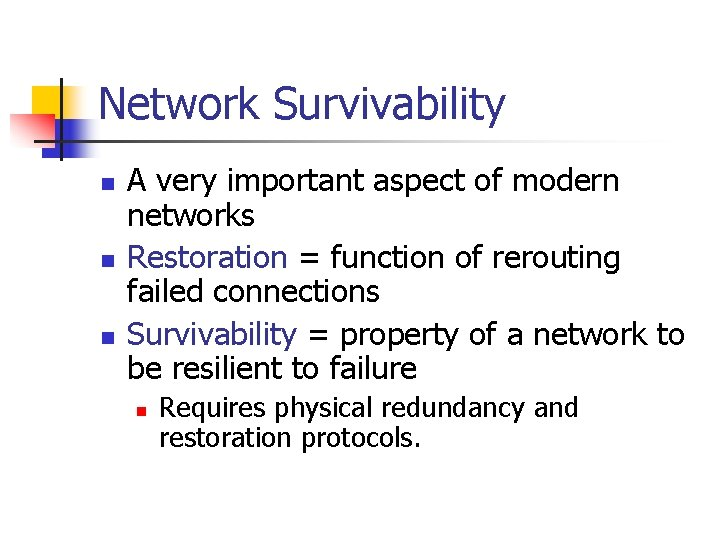Network Survivability n n n A very important aspect of modern networks Restoration = function of rerouting failed connections Survivability = property of a network to be resilient to failure n Requires physical redundancy and restoration protocols.Reactive / Proactive Reactive n n n A search is initiated to find a new lightpath which does not use the failed components after the failure happens. It can not guarantee successful recovery, Longer restoration time Proactive n Backup lightpaths are identified and resources are reserved at the time of establishing the primary lightpath itself. Taxonomy n n 100 percent restoration Faster recovery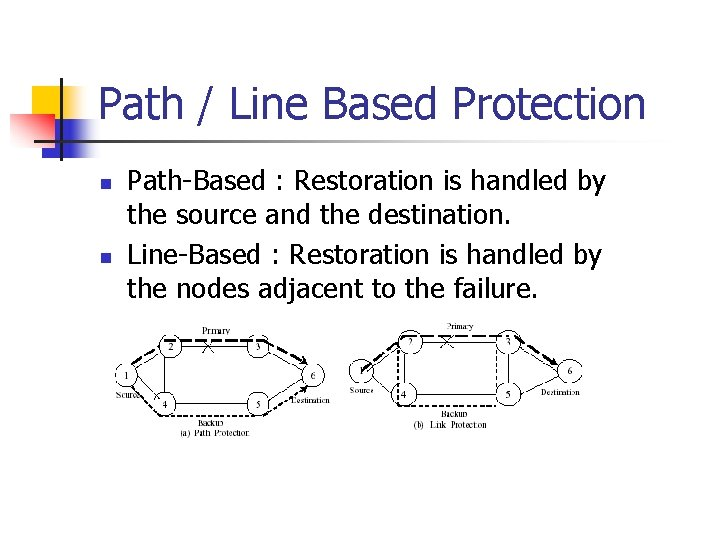Path / Line Based Protection n n Path-Based : Restoration is handled by the source and the destination. Line-Based : Restoration is handled by the nodes adjacent to the failure.Restoration Techniques n Dedicated Back (1+1, 1: 1) n n n One primary lightpath have one backup lightpath. Pros: simple for implementation and fast restoration Cons: waste of bandwidthRestoration Techniques /cont. n Backup Multiplexing (1: N) n n Allows two or more backup lightpaths to share a channel if the corresponding primary lightpaths do not fail simultaneously. Pros: better network utilization.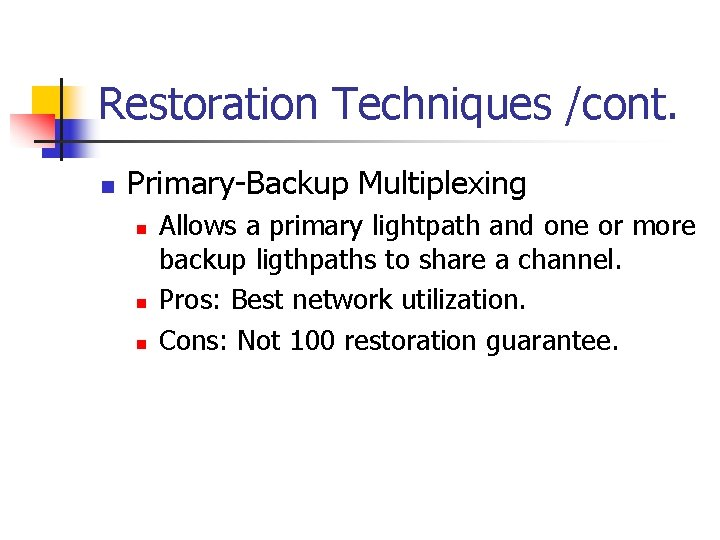Restoration Techniques /cont. n Primary-Backup Multiplexing n n n Allows a primary lightpath and one or more backup ligthpaths to share a channel. Pros: Best network utilization. Cons: Not 100 restoration guarantee.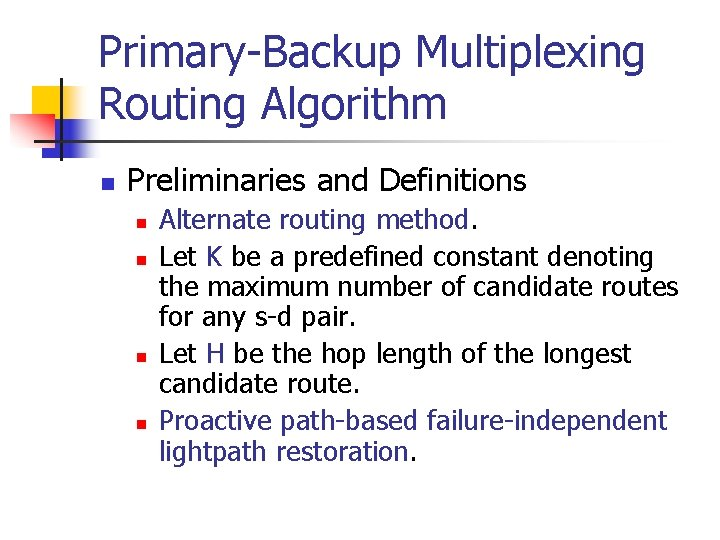Primary-Backup Multiplexing Routing Algorithm n Preliminaries and Definitions n n Alternate routing method. Let K be a predefined constant denoting the maximum number of candidate routes for any s-d pair. Let H be the hop length of the longest candidate route. Proactive path-based failure-independent lightpath restoration.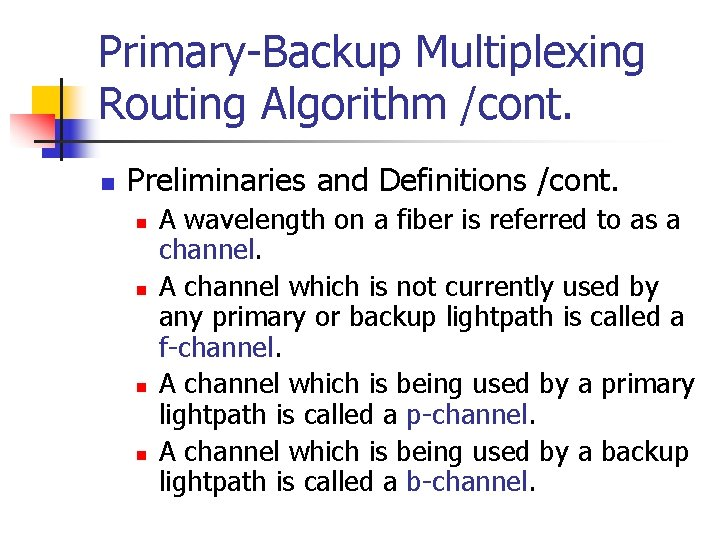Primary-Backup Multiplexing Routing Algorithm /cont. n Preliminaries and Definitions /cont. n n A wavelength on a fiber is referred to as a channel. A channel which is not currently used by any primary or backup lightpath is called a f-channel. A channel which is being used by a primary lightpath is called a p-channel. A channel which is being used by a backup lightpath is called a b-channel.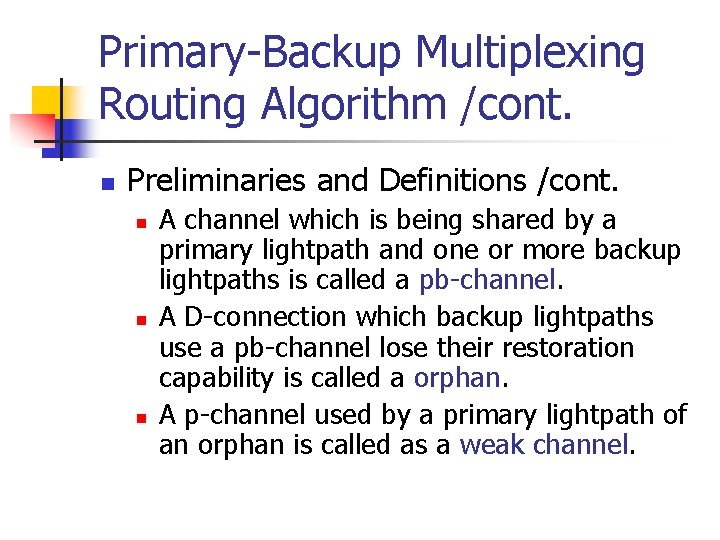Primary-Backup Multiplexing Routing Algorithm /cont. n Preliminaries and Definitions /cont. n n n A channel which is being shared by a primary lightpath and one or more backup lightpaths is called a pb-channel. A D-connection which backup lightpaths use a pb-channel lose their restoration capability is called a orphan. A p-channel used by a primary lightpath of an orphan is called as a weak channel.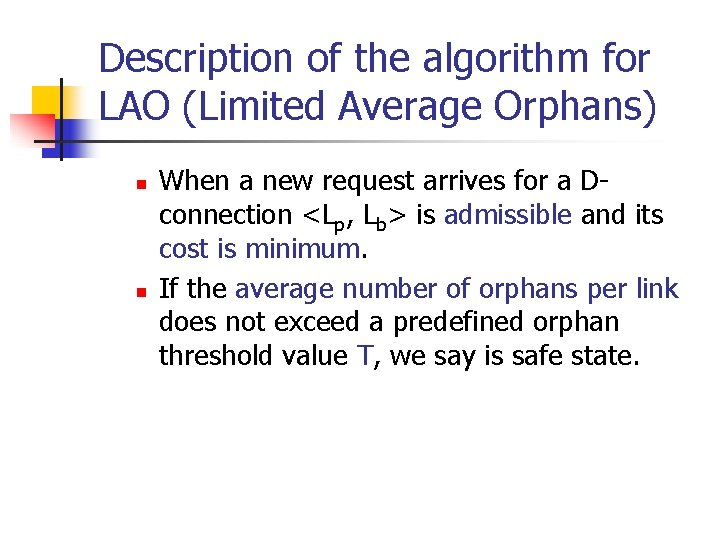Description of the algorithm for LAO (Limited Average Orphans) n n When a new request arrives for a Dconnection <Lp, Lb> is admissible and its cost is minimum. If the average number of orphans per link does not exceed a predefined orphan threshold value T, we say is safe state.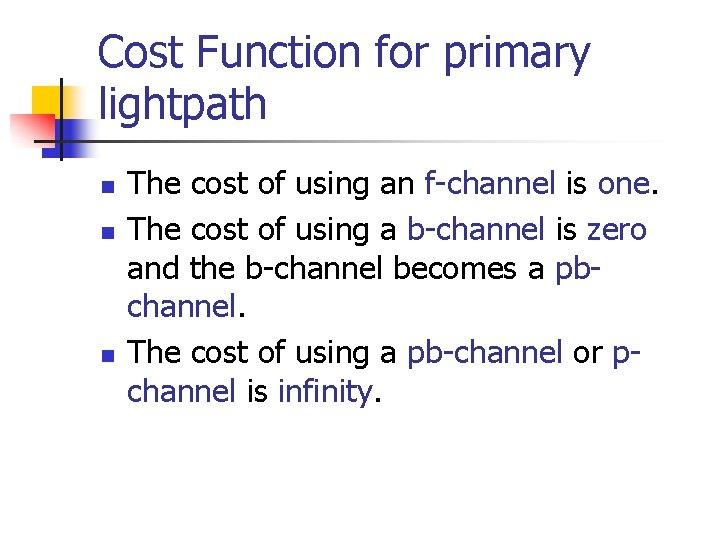Cost Function for primary lightpath n n n The cost of using an f-channel is one. The cost of using a b-channel is zero and the b-channel becomes a pbchannel. The cost of using a pb-channel or pchannel is infinity.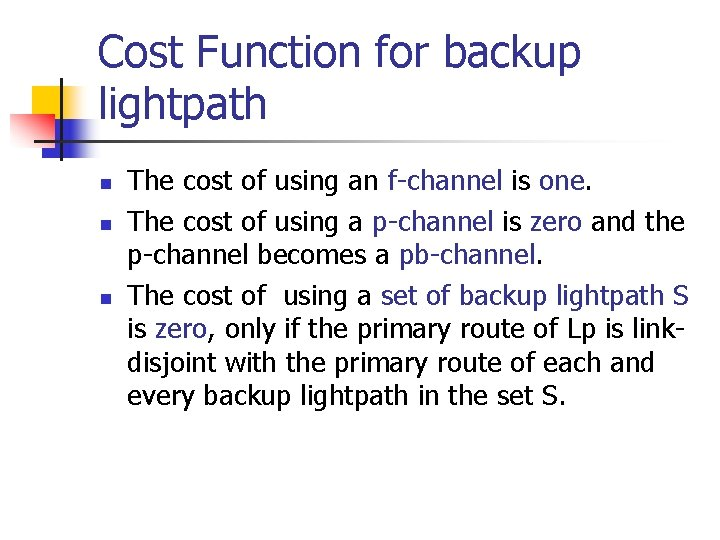Cost Function for backup lightpath n n n The cost of using an f-channel is one. The cost of using a p-channel is zero and the p-channel becomes a pb-channel. The cost of using a set of backup lightpath S is zero, only if the primary route of Lp is linkdisjoint with the primary route of each and every backup lightpath in the set S.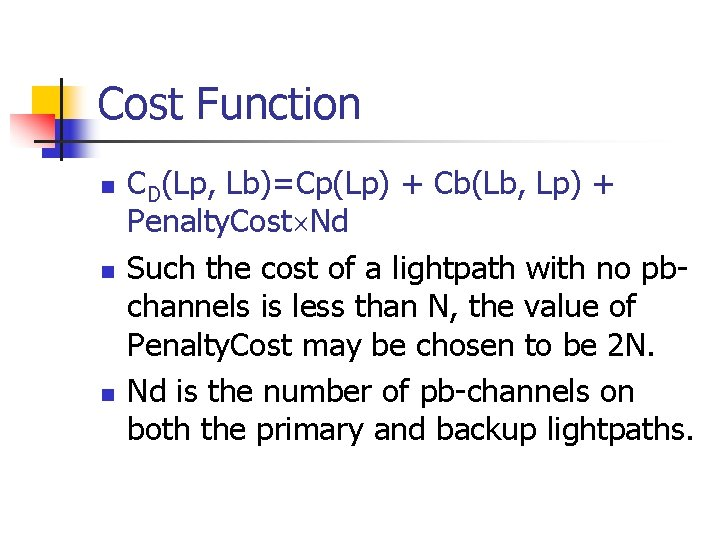Cost Function n CD(Lp, Lb)=Cp(Lp) + Cb(Lb, Lp) + Penalty. Cost Nd Such the cost of a lightpath with no pbchannels is less than N, the value of Penalty. Cost may be chosen to be 2 N. Nd is the number of pb-channels on both the primary and backup lightpaths.Admissibility Test function Test(Lp, Lb) : boolean begin x = X(Lp) y = Y(Lp, Lb) if Lorp + x + y > T then return false Lorp = Lorp + x + y return true end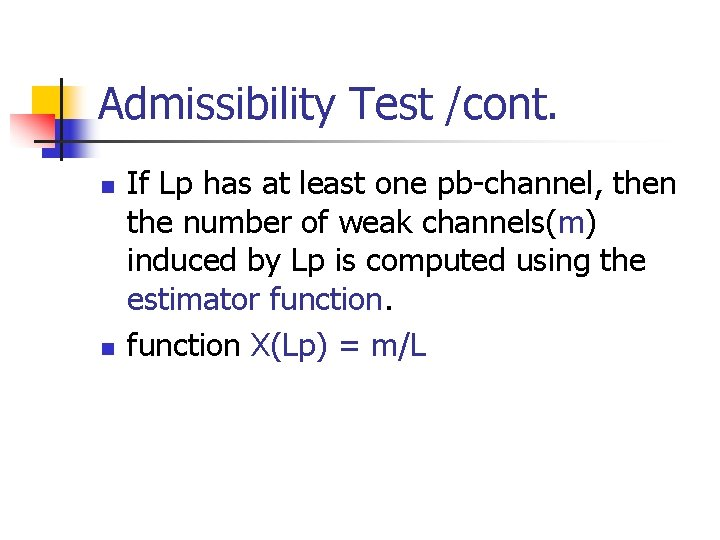Admissibility Test /cont. n n If Lp has at least one pb-channel, then the number of weak channels(m) induced by Lp is computed using the estimator function X(Lp) = m/LAdmissibility Test /cont. n n If the backup lightpath Lb has at least one pb-channel, then the channels used by the primary lightpath Lp are made weak. function Y(Lp, Lb) = h/L, where h is the hop length of Lp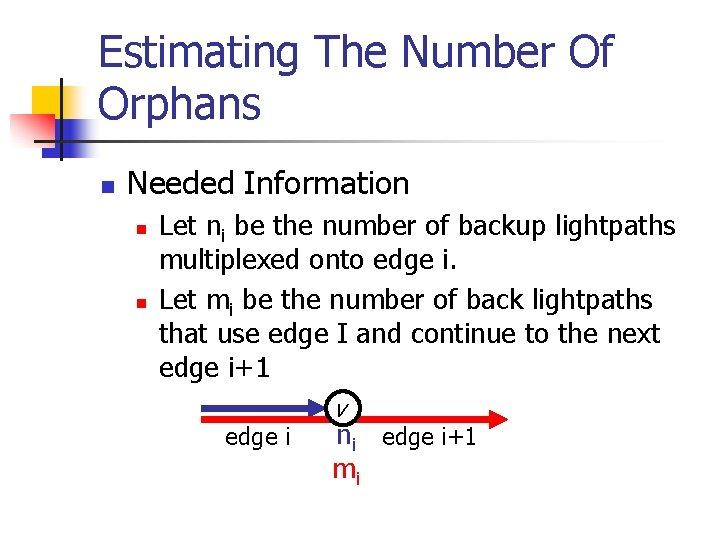Estimating The Number Of Orphans n Needed Information n n Let ni be the number of backup lightpaths multiplexed onto edge i. Let mi be the number of back lightpaths that use edge I and continue to the next edge i+1 edge i v ni edge i+1 mi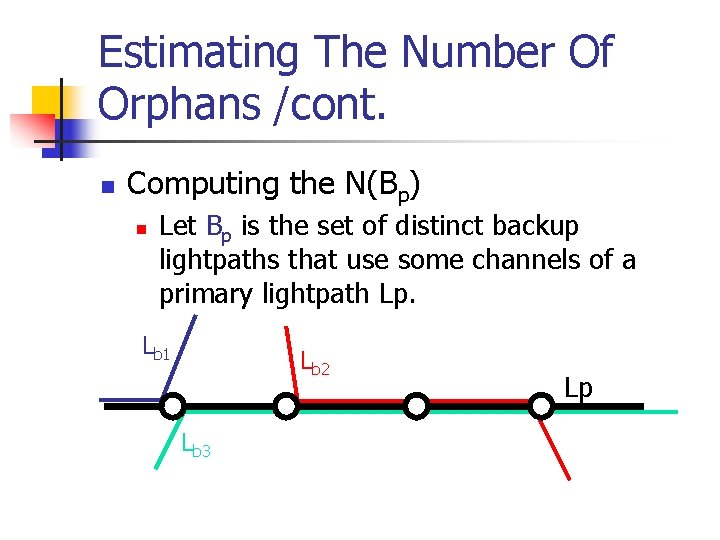Estimating The Number Of Orphans /cont. n Computing the N(Bp) n Let Bp is the set of distinct backup lightpaths that use some channels of a primary lightpath Lp. Lb 1 Lb 2 Lb 3 LpComputing the N(Bp) /cont. n Let ei, 0<i<H be the number of backup lightpaths that use edge i and do not use any of the edges 0 through i-1.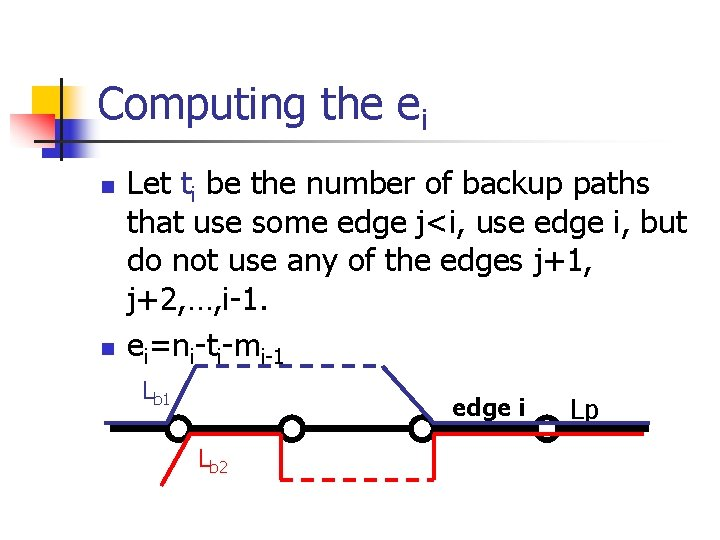Computing the ei n n Let ti be the number of backup paths that use some edge j<i, use edge i, but do not use any of the edges j+1, j+2, …, i-1. ei=ni-ti-mi-1 Lb 1 edge i Lb 2 Lp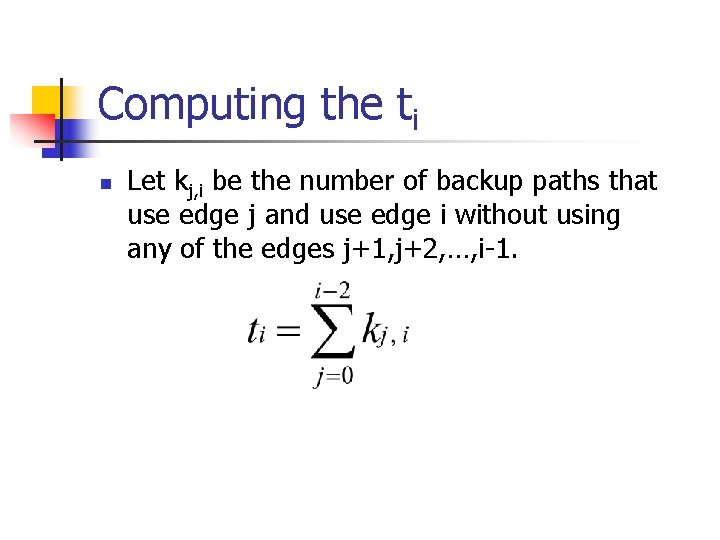Computing the ti n Let kj, i be the number of backup paths that use edge j and use edge i without using any of the edges j+1, j+2, …, i-1.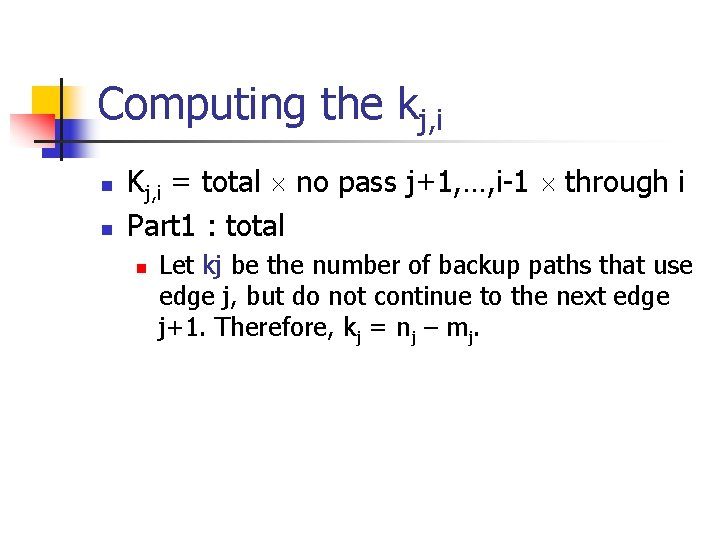Computing the kj, i n n Kj, i = total no pass j+1, …, i-1 through i Part 1 : total n Let kj be the number of backup paths that use edge j, but do not continue to the next edge j+1. Therefore, kj = nj – mj.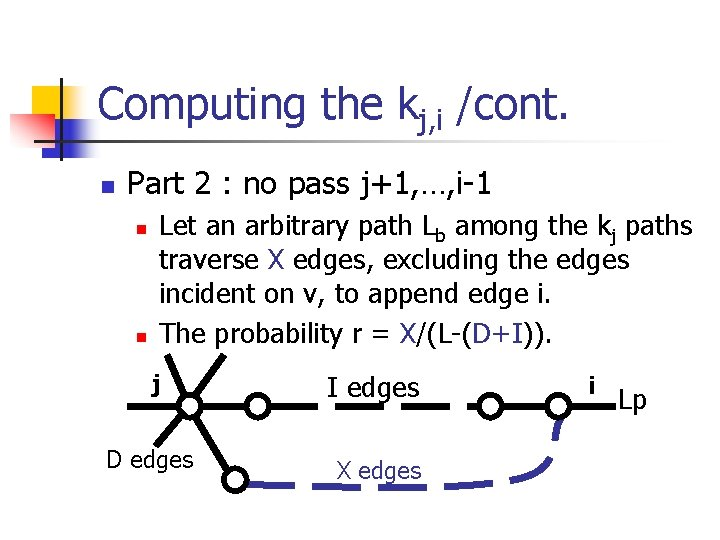Computing the kj, i /cont. n Part 2 : no pass j+1, …, i-1 n n Let an arbitrary path Lb among the kj paths traverse X edges, excluding the edges incident on v, to append edge i. The probability r = X/(L-(D+I)). j I edges D edges X edges i Lp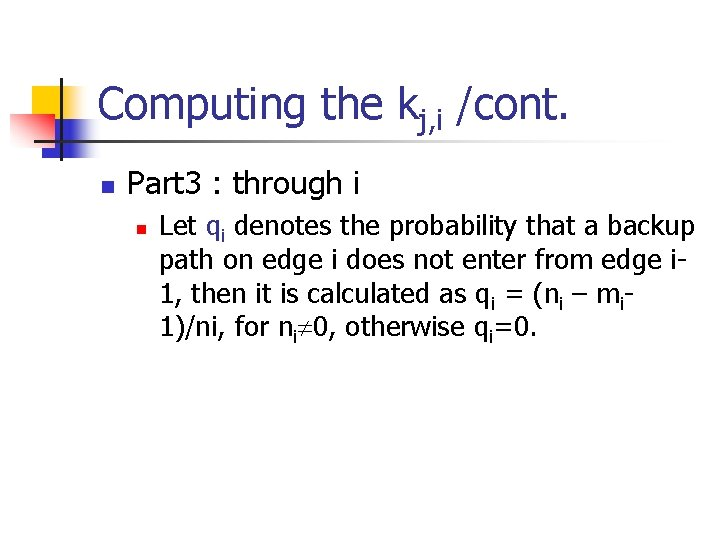Computing the kj, i /cont. n Part 3 : through i n Let qi denotes the probability that a backup path on edge i does not enter from edge i 1, then it is calculated as qi = (ni – mi 1)/ni, for ni 0, otherwise qi=0.Computing the Number of Weak Channels n Definition n A b-channel is said to cover a p-channel if the primary lightpath that uses the pchannel has a backup lightpath that uses the b-channel. Lp 3 1 5 2 4 6 Lb 7 8 9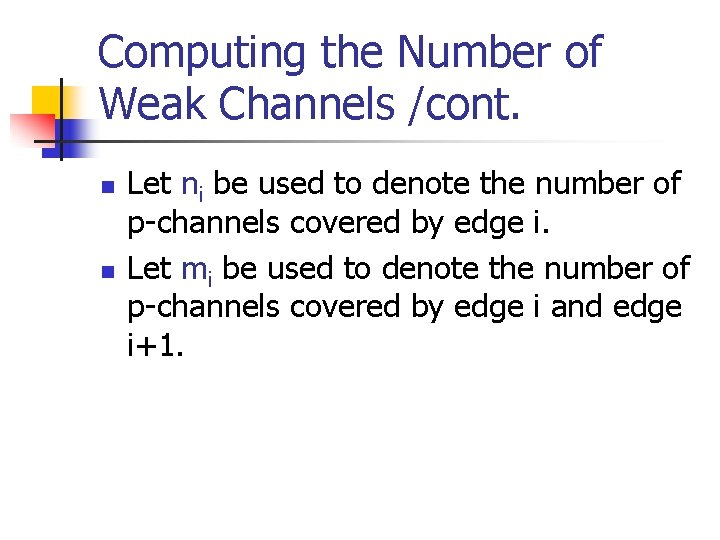Computing the Number of Weak Channels /cont. n n Let ni be used to denote the number of p-channels covered by edge i. Let mi be used to denote the number of p-channels covered by edge i and edge i+1.Estimate Function n function Estimate(Lp) : Given a path Lp with h edges and their n and m values. n Step 1) e 0=n 0 ; e 1=n 1 -m 0 ; val=e 0+e 1 k 0=n 0 -m 0 ; k 1=n 1 -m 1 S 0=k 0 ; S 1=k 0+k 1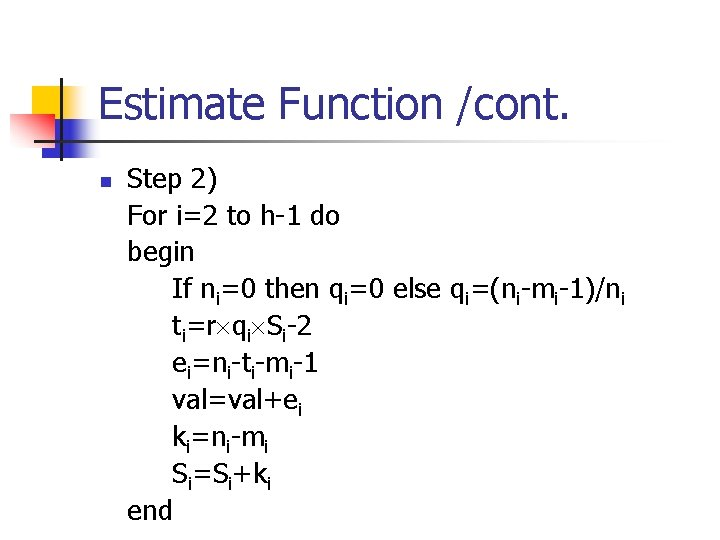Estimate Function /cont. n Step 2) For i=2 to h-1 do begin If ni=0 then qi=0 else qi=(ni-mi-1)/ni ti=r qi Si-2 ei=ni-ti-mi-1 val=val+ei ki=ni-mi Si=Si+ki end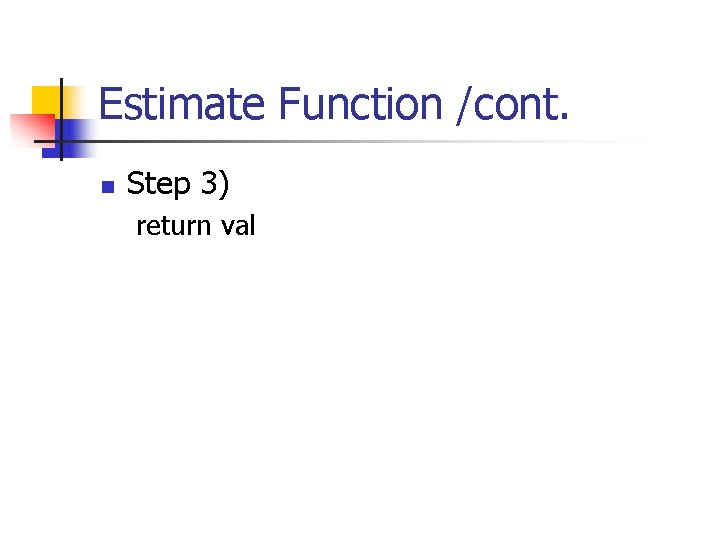Estimate Function /cont. n Step 3) return val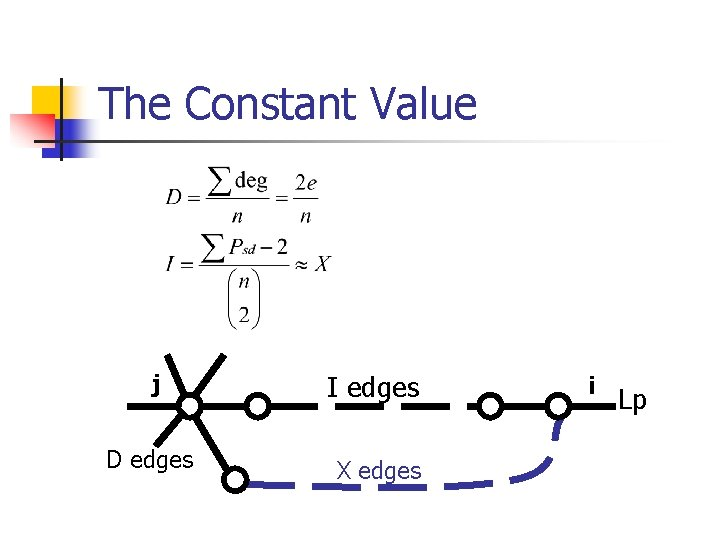The Constant Value j I edges D edges X edges i Lp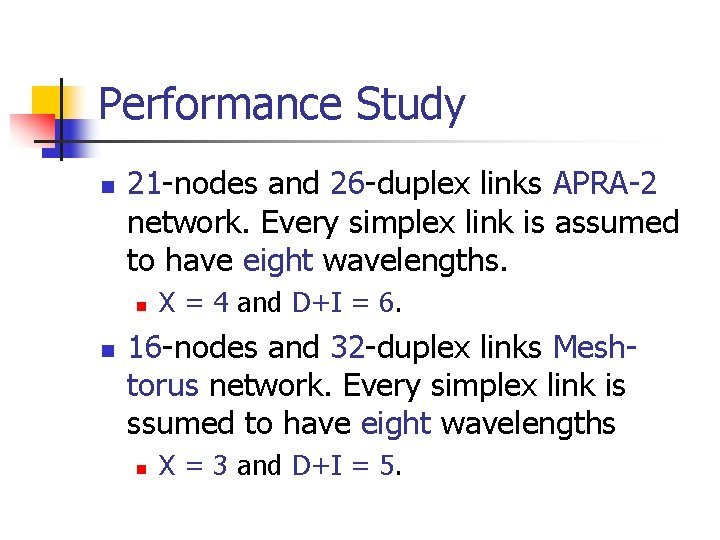Performance Study n 21 -nodes and 26 -duplex links APRA-2 network. Every simplex link is assumed to have eight wavelengths. n n X = 4 and D+I = 6. 16 -nodes and 32 -duplex links Meshtorus network. Every simplex link is ssumed to have eight wavelengths n X = 3 and D+I = 5.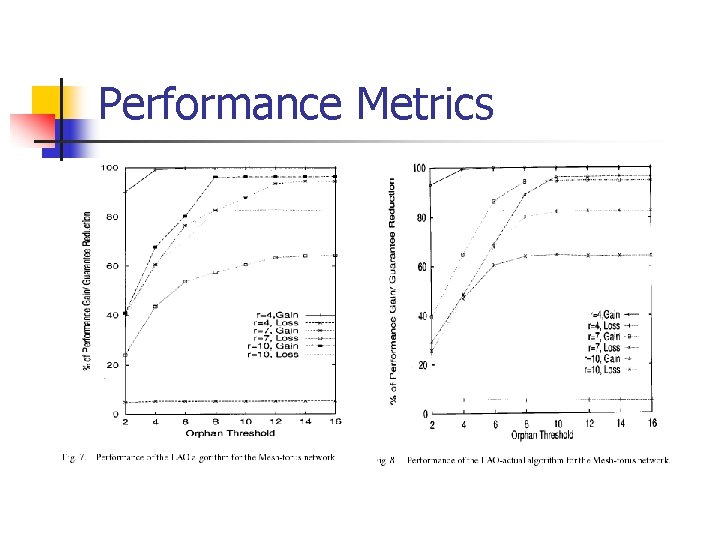Performance Metrics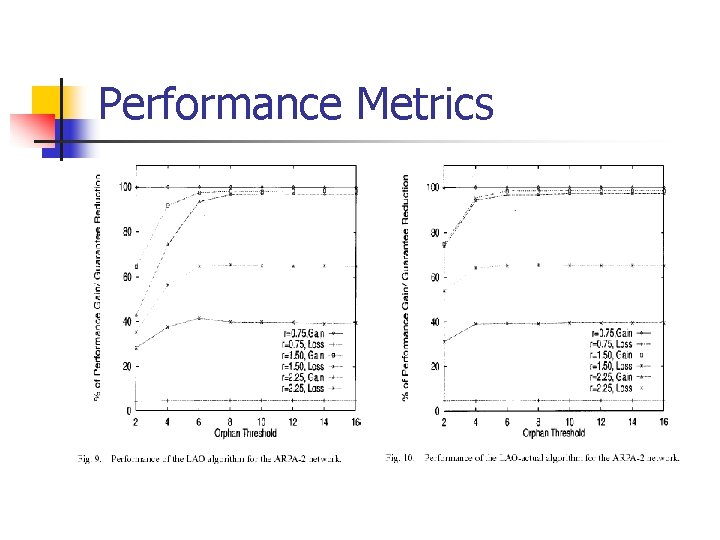Performance MetricsPerformance Metrics /cont.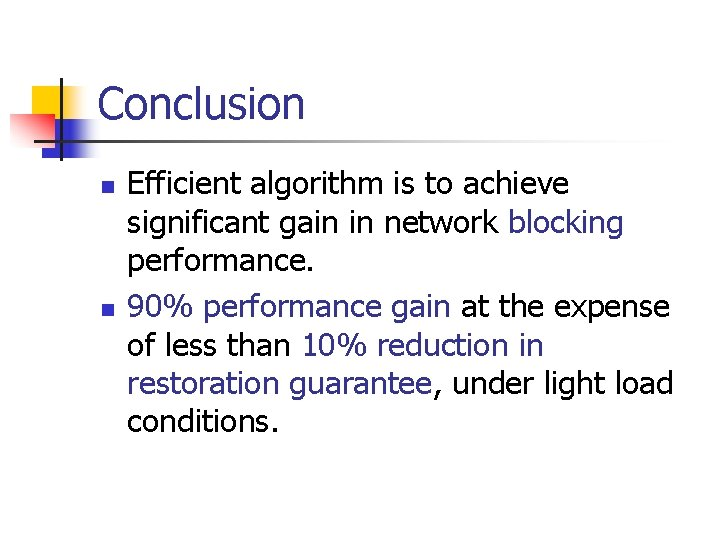Conclusion n n Efficient algorithm is to achieve significant gain in network blocking performance. 90% performance gain at the expense of less than 10% reduction in restoration guarantee, under light load conditions.